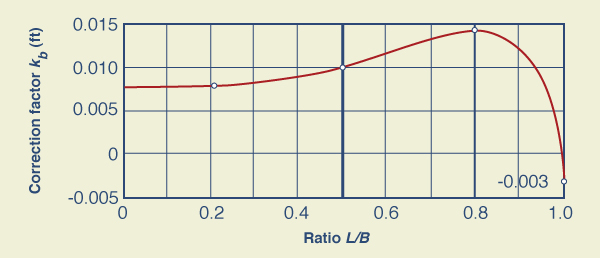Online Rectangular Weir Calculator

131028

The program online_rectangular_weir calculates the discharge through a rectangular weir (Fig. 1). To produce full contraction, the crest and sides of the weir plate are placed sufficiently far enough from the bottom and sides of the approach channel. The computation procedure follows Section 6 of Chapter 7 of the USBR Water Measurement Manual.Fig. 1  Rectangular weir schematic.

The Kindsvater-Carter formula for a rectangular weir, in U.S. Customary units, is:

 Q = Ce (L + kb) (H + 0.003)3/2 

in which Ce = effective coefficient of discharge; L = length of the weir crest, in ft, kb = a correction factor to obtain effective weir length, in ft; H = head measured above the weir crest, in ft; and Q = discharge, in cfs. The value B is the average width of the approach channel.

The correction factor kb is a function of the ratio L/B, as shown in Fig. 2.Fig. 2  Correction factor kb to obtain effective weir length.

The effective coefficient of discharge Ce includes effects of relative depth and relative width of the approach channel. It is a function of H/P and L/B, as shown in Fig. 3.Fig. 3  Values of Ce as a function of H/P and L/B.

Given H, L, B and P, and the ratios H/P and L/B, the computation proceeds with the following steps:

1. The correction factor kb is calculated using Fig. 2.

2. The effective coefficient of discharge Ce is calculated using Fig. 3.

3. The discharge Q is calculated using Eq. 1.

The rectangular weir equation (Eq. 1) is subject to the following restrictions:

1. The calibration relationships (Figs. 2 and 3) were developed with rectangular approach flow. For applications with other flow section shapes, the average width of the flow section for each head should be used as B  to calculate discharges.

2. The head H  should be at least 0.2 ft (0.061 m).

3. The crest height P ≥ 4 in (0.3333 ft, or 0.1015 m).

4. The crest length L ≥ 6 in (0.5 ft, or 0.1524 m).

5. The ratio H/P ≤ 2.4.

6. The water surface elevation in the downstream channel should be at least 2 in (5 cm, or 0.05 m) below the weir crest.

 131103